Next: Orbital motion Up: Oscillatory motion Previous: Worked example 11.5: Gravity

## Worked example 11.6: Oscillating disk

Question: A uniform disk of radius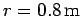and mass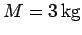is freely suspended from a horizontal pivot located a radial distance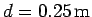from its centre. Find the angular frequency of small amplitude oscillations of the disk.

Answer: The moment of inertia of the disk about a perpendicular axis passing through its centre is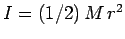. From the parallel axis theorem, the moment of inertia of the disk about the pivot point is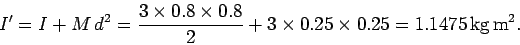The angular frequency of small amplitude oscillations of a compound pendulum is given by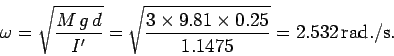Hence, the answer is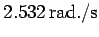.

Richard Fitzpatrick 2006-02-02